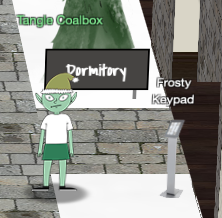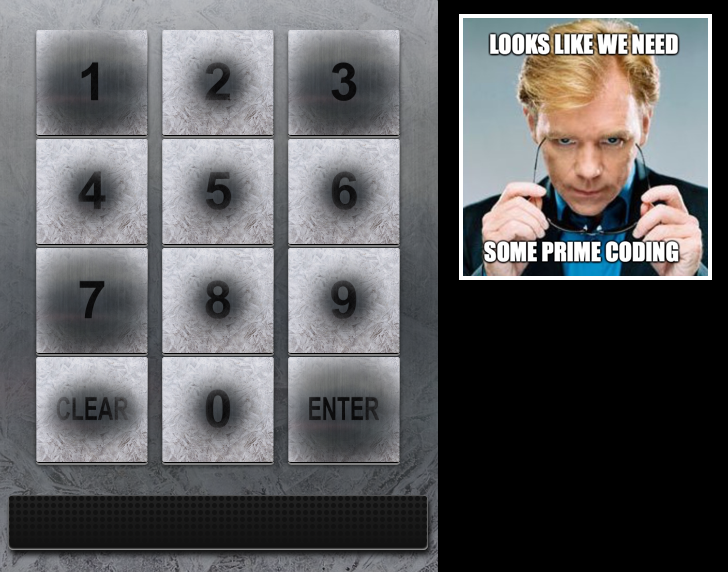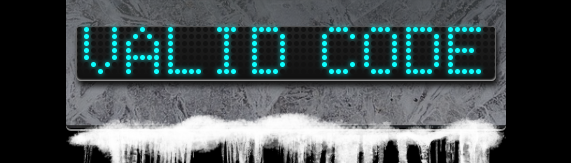# Tangle Coalbox - Frosty Keypad#Objective: Splunk

## Request#

Hey kid, it's me, Tangle Coalbox.
I'm sleuthing again, and I could use your help.
Ya see, this here number lock's been popped by someone.
I think I know who, but it'd sure be great if you could open this up for me.
I've got a few clues for you.

1. One digit is repeated once.
2. The code is a prime number.
3. You can probably tell by looking at the keypad which buttons are used.

## Resources#

• "One digit is repeated once, it's prime, and you can see which keys were used"

## Solution#Based on the hints and the keypad image we see that 1, 3, 7, and 'enter' are the keys that are used most frequently. Since one of the 3 digits is also repeated once the total code has a length of 4. This limits the options to permutations of the following 3 number sets: [1, 1, 3, 7], [1, 3, 3, 7], and [1, 3, 7, 7]. Finally our 4-digit number needs to be prime. To help solve this we can use the following `find_code.py` Python script.

``````#!/usr/bin/env python3
"""2019 SANS Holiday Hack Challenge - Frosty Keypad."""
import itertools

def is_prime(number):
"""Verify if a number is a prime."""
return 2 in [number, 2**number % number]

def main():
"""Execute."""
digit_sets = [
['1', '1', '3', '7'],
['1', '3', '3', '7'],
['1', '3', '7', '7']
]

primes = []

for digits in digit_sets:
for subset in itertools.permutations(digits):
val = int(''.join(subset))
if is_prime(val) and val not in primes:
primes.append(val)
print(f'{val} is a prime number')

if __name__ == "__main__":
main()
``````

The script generates a manageable list of 5 possible candidates.

``````1373 is a prime number
1733 is a prime number
3137 is a prime number
3371 is a prime number
7331 is a prime number
``````Looks like `7331` is the correct code!

Code: `7331`